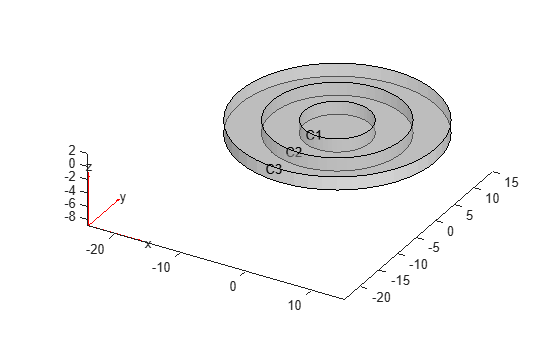Documentation

This is machine translation

Mouseover text to see original. Click the button below to return to the English version of the page.

findStructuralIC

Find initial displacement and velocity assigned to geometric region

Syntax

``sica = findStructuralIC(structuralmodel.InitialConditions,RegionType,RegionID)``

Description

example

````sica = findStructuralIC(structuralmodel.InitialConditions,RegionType,RegionID)` returns the initial displacement and velocity assigned to the specified region.```

Examples

collapse all

Find the initial displacement and velocity assigned to the cells of a 3-D geometry.

Create a transient dynamic model for a 3-D problem.

`structuralmodel = createpde('structural','transient-solid');`

Create the geometry consisting of the three nested cylinders and include it in the model. Plot the geometry.

```gm = multicylinder([5 10 15],2); structuralmodel = createpde('structural','transient-solid'); structuralmodel.Geometry = gm; pdegplot(structuralmodel,'CellLabels','on','FaceAlpha',0.5)```Set the initial conditions for each cell. When you specify only the initial velocity or initial displacement, `structuralIC` assumes that the omitted parameter is zero.

```structuralIC(structuralmodel,'Displacement',[0;0;0],'Velocity',[0;0;0],'Cell',1); structuralIC(structuralmodel,'Displacement',[0;0.1;0],'Cell',2); structuralIC(structuralmodel,'Velocity',[0;0.2;0],'Cell',3);```

Check the initial condition specification for cell 1.

`SICACell1 = findStructuralIC(structuralmodel.InitialConditions,'Cell',1)`
```SICACell1 = GeometricStructuralICs with properties: RegionType: 'Cell' RegionID: 1 InitialDisplacement: [3x1 double] InitialVelocity: [3x1 double] ```
`SICACell1.InitialDisplacement`
```ans = 3×1 0 0 0 ```
`SICACell1.InitialVelocity`
```ans = 3×1 0 0 0 ```

Use a static solution as an initial condition for a dynamic structural model. Check and plot the initial displacement.

Create a static model.

`staticmodel = createpde('structural','static-solid');`

Create the geometry and include it in the model. Plot the geometry.

```gm = multicuboid(0.06,0.005,0.01); staticmodel.Geometry = gm; pdegplot(staticmodel,'FaceLabels','on','FaceAlpha',0.5) view(50,20)```Specify the Young's modulus, Poisson's ratio, and mass density.

```structuralProperties(staticmodel,'YoungsModulus',210E9, ... 'PoissonsRatio',0.3,... 'MassDensity',7800);```

Apply the boundary condition and static load.

```structuralBC(staticmodel,'Face',5,'Constraint','fixed'); structuralBoundaryLoad(staticmodel,'Face',3,'SurfaceTraction',[0;1E6;0]); generateMesh(staticmodel,'Hmax',0.02); Rstatic = solve(staticmodel);```

Create a dynamic model and assign geometry.

```dynamicmodel = createpde('structural','transient-solid'); gm = multicuboid(0.06,0.005,0.01); dynamicmodel.Geometry = gm;```

Apply the boundary condition.

`structuralBC(dynamicmodel,'Face',5,'Constraint','fixed');`

Specify the initial condition using the static solution.

```generateMesh(dynamicmodel,'Hmax',0.02); structuralIC(dynamicmodel,Rstatic)```
```ans = NodalStructuralICs with properties: InitialDisplacement: [113x3 double] InitialVelocity: [113x3 double] ```

Check the initial condition specification for `dynamicmodel`.

`sica = findStructuralIC(dynamicmodel.InitialConditions,'Cell',1)`
```sica = NodalStructuralICs with properties: InitialDisplacement: [113x3 double] InitialVelocity: [113x3 double] ```

Plot the `z`-component of the initial displacement.

```pdeplot3D(dynamicmodel,'ColorMapData',sica.InitialDisplacement(:,3)) title('Initial Displacement in the Z-direction')```Input Arguments

collapse all

Initial conditions of a transient structural model, specified as the `InitialConditions` property of a `StructuralModel` object.

Geometric region type, specified as `'Face'`, `'Edge'`, or `'Vertex'` for a 2-D model or 3-D model, or `'Cell'` for a 3-D model.

Data Types: `char`

Geometric region ID, specified as a vector of positive integers. Find the region IDs using `pdegplot`, as shown in Create Geometry and Remove Face Boundaries or STL File Import.

Data Types: `double`

Output Arguments

collapse all

Structural initial condition for a particular region, returned as a `GeometricStructuralICs` or `NodalStructuralICs` object. For details, see GeometricStructuralICs Properties and NodalStructuralICs Properties.

Introduced in R2018a

Partial Differential Equation Toolbox DocumentationGet trial now